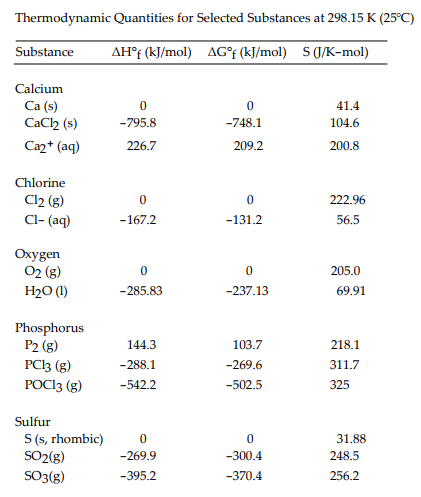# Problem: Use the table below to answer the questions that follow.The value of ΔG° at 25 °C for the oxidation of solid elemental sulfur to gaseous sulfur dioxide,S(s, rhombic) + O2(g) --&gt; SO2(g) is __________ kJ/mol.a. -300.4 kJ/molb. -269.9 kJ/molc. +300.4 kJ/mold. +269.9 kJ/mole. -370.4 kJ/mol

###### FREE Expert Solution

We’re being asked to determine the value of ΔG° at 25 °C for the oxidation of solid elemental sulfur to gaseous sulfur dioxide:

S(s, rhombic) + O2(g) → SO2(g)

We can use the following equation to solve for ΔG˚rxn

96% (350 ratings)###### Problem Details

Use the table below to answer the questions that follow.The value of ΔG° at 25 °C for the oxidation of solid elemental sulfur to gaseous sulfur dioxide,

S(s, rhombic) + O2(g) --> SO2(g)

is __________ kJ/mol.

a. -300.4 kJ/mol
b. -269.9 kJ/mol
c. +300.4 kJ/mol
d. +269.9 kJ/mol
e. -370.4 kJ/mol